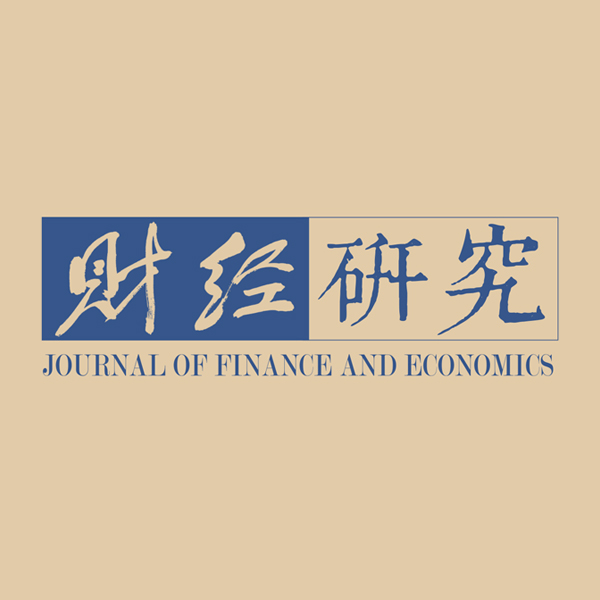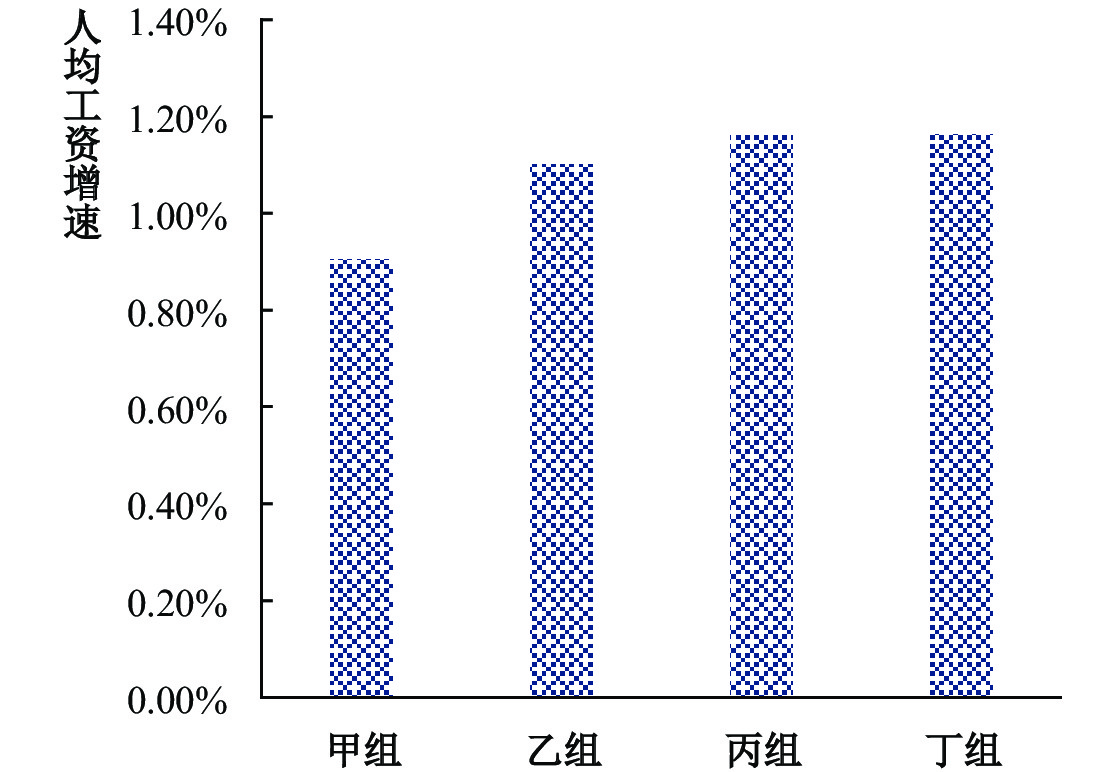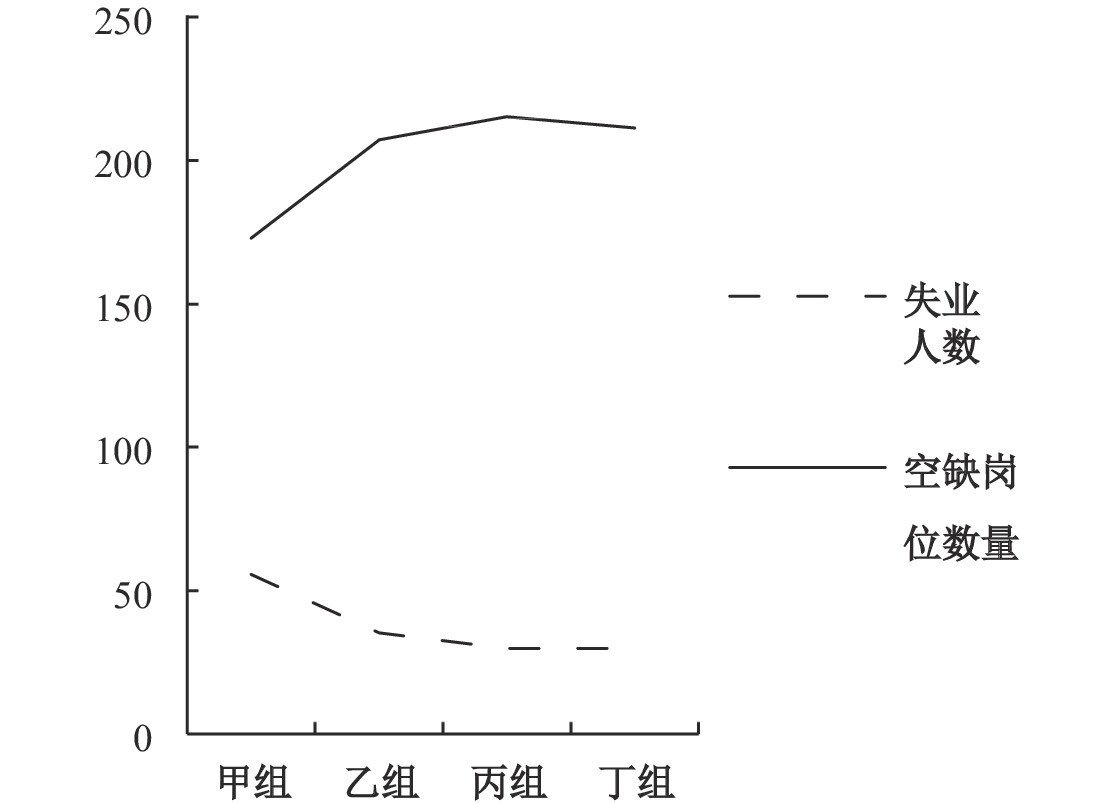﻿ 工作搜寻范围扩大对劳动力市场的影响《财经研究》
2020第46卷第9期

1. 华南师范大学 经济与管理学院，广东 广州 510006;
2. 澳门城市大学 商学院，澳门 999078

Impact of the Expansion of Job Search Scope on the Labor Market
Chen Yiting1, Dong Zhiqiang1,21. School of Economics & Management，South China Normal University，Guangzhou 510006，China;
2. Faculty of Business，City University of Macau，Macau 999078，China
Key words: search scope of workers    ABM    labor market

ABM劳动力市场模型与传统的数理模型相比，有其自身的独特性。以往的数理模型大多以单一工人的行为来代表全体工人的行为，对企业的做法也是如此。然而，这种做法忽略了工人之间行为的相互影响：当一个失业工人获得了一个就业机会，这有可能意味着另一个失业工人失去了就业机会。ABM模型是由数量众多的主体构成的，如本文的劳动力市场模型中包含了大量的工人主体和企业主体，工人之间、企业之间、工人和企业之间的相互影响都可以在模型中体现出来。与传统的代表性单主体模型不同，ABM模型中众多主体之间同时进行的互动和交易构成了一个具有复杂性的动态系统，这可以为劳动力市场的研究提供一些新的理解。

ABM劳动力市场模型中的主体也有着非常独特的行为模式。在传统的数理模型中，工人主体对自身的收益和成本进行精确、复杂的计算，并且根据计算结果直接作出最优反应。但是行为经济学已经向人们揭示，经济行为主体往往是在信息不完全、理性计算不充分的情况下，作出偏离最优反应的决策。而ABM模型的优势之一在于，它可以很方便地对有限理性主体的行为进行刻画。如本文的ABM劳动力市场模型，工人所能够获取的信息仅仅局限于其搜寻范围，其关于成本和收益的计算也只依赖于非常有限的信息。模型中的工人还可以依据以往的历史经历来调整自己的行为，但是也只是理性程度非常低的适应性调整，并非经过周密计算的最优反应。因此，相较于假设行为主体的完全理性的模型，ABM劳动力市场模型对行为主体有限理性的刻画更加具有现实性。

$I$
$J$
，地理位置是随机分布的。时间是离散的，即
$t = 0,1,2 \cdots \cdots$
，而企业和工人的匹配是商品生产的必要条件。Fagiolo等（2004）在其模型中考虑了技术进步的情况，但是生产效率的进步并不是本文关注的重点，为了简化模型，假设企业之间和工人之间没有生产效率的差别，也不会发生变化，并且1个就业工人每期生产1单位商品。在模型中只存在唯一1种商品。

（一）企业

$i$
$t$

 ${y_{it}} = {n_{it}}$ (1)

${{\rm{y}}_{it}}$

$i$
$t$

${n_{it}}$

$i$

$i$

 ${\pi _{it}} = {p_t}{y_{it}} - {n_{it}}{w_{it}}$ (2)

${p_t}$

$t$

${w_{it}}$

$i$
$t$

$i$
$t$

${C_{it}}$
。企业的盈利状况将影响到下一期的资产数量，可表达为：

 ${C_{i,t + 1}} = {C_{it}} + {\pi _{it}}$ (3)

${\pi _{it}} > 0$
）时，将实现资产增长，反之则出现资产萎缩。模型考虑了长期亏损企业的出清。当企业的资产数量
${C_{it}} < 0$

（二）工人

$j$

${r_j}$
，他所能获取的岗位信息仅仅局限于搜寻范围之内。工人为了实现收益最大化的目标，他对处于搜寻范围内的就业机会进行收益与成本的权衡，并给出评价。然后，依据该评价来决定是否前往应聘和前往应聘的先后顺序。工人
$j$

$i$

${V_{ji}}$

 ${V_{ji}} = {w^s_{it}}{a_j} - {c_{ji}}$ (4)
 ${c_{ij}} = {\varpi _t}{d_{ij}}$ (5)
 ${\varpi _t} = \kappa {\bar w_{t - 1}}$ (6)

$w_{it}^s$

$i$

${a_j}$

$j$

${c_{ji}}$

$j$

$i$

${d_{ij}}$

${\varpi _t}$

${\bar w_{t - 1}}$

$j$

${V_{ji}}$

${b_t}$
，因此工人可以在就业和失业之间进行权衡。但是由于所有工人都处在搜寻工作阶段，因此无法预知本期的人均工资水平，也无法预知本期的失业保险金额，所以只能以上一期失业保险金额
${b_{t - 1}}$

${V_{ji}}$

${b_{t - 1}}$

（三）工资制定

$w_{it}^s$
，企业实际的工资需要与工人们进行谈判而形成。模型假设，每个工人也都具有一个心理期望工资水平
${w^s_{jt}}$
。企业与前来应聘成功的工人们进行工资谈判，最后确定该企业的工资水平，工资制定过程具体表达为：

 ${w_{it}}^{} = {w^s_{it}}{\sigma _{it}} + \frac{1}{{{n_{it}}}}\sum\limits_{j = 1}^{{n_{it}}} {w^s_{jt}} (1 - {\sigma _{it}})$ (7)
 ${\sigma _{it}} = \frac{{{n_{it}}}}{{{N_{it}}}}$ (8)

${\sigma _{_{it}}}$

$i$
$t$

$i$
$t$

${w_{it}}$

$w_{it}^s$

$\dfrac{1}{{{n_{it}}}}\sum\limits_{j = 1}^{{n_{it}}} {w_{jt}^s}$

${\sigma _{{\rm{it}}}}$

$1{\rm{ - }}{\sigma _{{\rm{it}}}}$

${\sigma _{{\rm{it}}}}$

${n_{it}}$

${N_{it}}$

$i$

$j$

$\theta \left( {0 < \theta < 1} \right)$

（四）消费市场

 ${Y_t} = \sum\limits_{i = 1}^I {{y_{it}}}$ (9)

 ${\bar w_t} = \frac{{\sum\limits_{j = 1}^J {{w_{jt}}} }}{{{{\sum\limits_{{\rm{i}} = 1}^I {{n_{it}}} }_{}}}}$ (10)

${b_t}$
。该失业保险源于就业工人的转移支付，将作为失业工人下一期的搜寻资源，并不用于本期的消费。失业保险与本期的人均工资水平
${\bar w_t}$

 ${b_t} = \mu {\bar w_t}$ (11)

${b_t}$
，这就意味着无论工人在本期中是就业的还是失业的，他在下一期的搜寻资源都是相等的。因此，本期的工资总和中能用于消费的金额为
$\sum\limits_{j = 1}^J {{w_{jt}} - {b_t}J}$

$\sum\limits_{j = 1}^J {{w_{jt}} - {b_t}J} + {b_{t - 1}}J$
。将本期的消费总金额除以总产量即可得到单位商品的价格水平：

 ${p_t} = \frac{{\sum\limits_{j = 1}^J {{w_{jt}} - {b_t}J + {b_{t - 1}}J} }}{{{Y_t}}}$ (12)

（五）动态调整

 ${\text{企业：}}{w^s}_{i,t + 1} = \left\{ \begin{array}{l} w_{it}^s(1 + {\xi _1}){\rm{ , }} \; {\xi _1} \in {\rm{(0,3\% ) \; if \; }}\dfrac{{{n_{it}}}}{{{N_{it}}}} < \eta \;{\rm{ or }}\;\dfrac{{{n_{i,t - 1}}}}{{{N_{i,t - 1}}}} < \eta {\rm{ }}\\ w_{it}^s(1 - {\xi _1}){\rm{ , }} \; {\xi _1} \in (0,3{\rm{\% }})\;{\rm{ if }}\;\dfrac{{{n_{it}}}}{{{N_{it}}}} \geqslant \eta \;{\rm{ and }}\;\dfrac{{{n_{i,t - 1}}}}{{{N_{i,t - 1}}}} \geqslant \eta \end{array} \right.$ (13)
 ${\text{工人：}}{w^s}_{j,t + 1} = \left\{ \begin{array}{l} {w^s}_{jt}(1 + {\xi _2}){\rm{, }} \; {\xi _2} \in {\rm{(0,3\% ) \; if \; }}{s_{jt}} = 1\;{\rm{ and }}\;{s_{j,t - 1}} = 1\\ {w^s}_{jt}(1 - {\xi _2}){\rm{, }} \; {\xi _2} \in {\rm{(0,3\% ) \; if\; }}{s_{jt}} = 0\;{\rm{ or }}\;{s_{j,t - 1}} = 0{\rm{ }} \end{array} \right.$ (14)

$i$

$\eta$
$0 \leqslant \eta \leqslant 1$
）时，为了缩减成本，企业会把下一期的期望工资水平
${w^s}_{i,t + 1}$

${\xi _1}$

${s_{jt}}$

$j$
$t$

$j$

${\xi _2}$

 ${N_{i,t + 1}} = \left\{ \begin{array}{l} {N_{it}} + 1\;{\rm{ if }}\;{\pi _{it}} > {\pi _{i,t - 1}}\\ {N_{it}} - 1\;{\rm{ if }}\;{\pi _{it}} < {\pi _{i,t - 1}} \end{array} \right.$ (15)

（六）总结图 1 劳动力市场模型子程序流程图

 变量 含义 初始取值 $I$ 企业数量 50 $J$ 工人数量 500 $\theta$ 工人对实际工资的接受底线 50% $\eta$ 企业对招工比例的满意程度 1 ${a_j}$ 工人应聘的成功概率 $\begin{array}{l}P({a_j} = 70{\rm{\% } }) = P({a_j} = 80{\rm{\% } }) = P({a_j} = 90{\rm{\% } }) = \dfrac{1}{3}\end{array}$ $\kappa$ 人均工资对单位移动成本的影响系数 2.25% $\mu$ 人均工资对失业保险的影响系数 45% ${N_{i1}}$ 初期企业岗位数量 服从 $N(\dfrac{I}{J},10)$ 分布 $w_{i1}^s$ 初期企业期望工资 服从 $N(100,10)$ 分布 $w_{j1}^s$ 初期工人期望工资 服从 $N(100,10)$ 分布 ${C_{i1}}$ 初期资产存量 100 ${r_j}$ 搜寻范围半径 6图 2 拟合的菲利普斯曲线、贝弗里奇曲线和奥肯曲线

（一）实验组设置

 实验组名称 甲组 乙组 丙组 丁组 搜寻范围取值 r=6 r=7 r=8 r=9

（二）失业与收入分析图 3 各实验组的失业率和总产量图 4 不同实验组平均每期的人均工资增速

（三）不同类型的失业分析

 失业类型 失业原因 A 搜寻范围内无企业或空缺岗位 B 就业的期望收益低于失业 C 因搜寻资源不足而中止搜寻 D 每次前往应聘，却都失败 E 应聘成功，但对工资谈判结果不满意

 失业类型 甲组 乙组 丙组 丁组 A 6.79% 3.11% 1.58% 0.89% B 0.00% 0.07% 0.44% 0.80% C 0.32% 0.69% 0.99% 1.16% D 3.15% 1.62% 0.71% 0.33% E 0.87% 1.57% 2.26% 2.79%

A类失业指的是工人在其搜寻范围内无法搜寻到企业或者空缺的岗位，从而被迫失业。这类失业本质上是由于工人搜寻范围的局限所导致的，因此从实验结果可以看出，随着工人搜寻范围的扩大，工人获得更多的就业机会。而此类失业率将逐步减少，并且效果非常明显。因此，此类失业率对总失业的下降有着重要的贡献。

B类失业指的是工人在前往应聘之前，其预估的就业收益低于失业保险而选择失业，从而主动失业。实验结果表明，随着搜寻范围的扩大，此类失业率会略微地上升。原因在于，新增加的就业机会与工人距离都比较远，这将增加其应聘的成本，使得预估的就业收益可能低于社会提供的失业保险，从而增加工人主动选择失业的概率。

C类失业指的是工人在搜寻过程中因搜寻资源不足而导致的失业。实验表明，此类失业将随着搜寻范围的扩大而增加，其原因与B类失业相似，也是由于搜寻范围的扩大而带来的应聘成本的提高，导致搜寻资源在工作搜寻过程中成为更明显的约束。

D类失业指的是工人虽然每次都有机会和资源前往应聘，但是在搜寻所处范围内全部空缺岗位之后，仍然遭到众多企业的拒绝而最终失业。此类失业在搜寻范围扩大的过程中将逐渐减少。虽然工人单次应聘的成功率并不直接与搜寻范围相关，而这是外生给定且固定不变的，但是搜寻范围的扩大将增加工人的就业机会，使其可以应聘更多的岗位，从而减少最终失业的概率。也有部分原因在于，工人搜寻范围的扩大增加其应聘成本，使得此类失业转为B、C类失业。

E类失业是企业和工人进行工资谈判之后，工人认为谈判所形成的工资低于自己的预期水平，从而选择主动失业。实验表明，此类失业将随着搜寻范围的扩大而增加。原因在于，当工人连续多期地处于就业状态时，其期望工资水平将得到提升，从而也更加容易发生此类失业。搜寻范围的扩大在改善了工人就业环境的同时，也提升了其预期的工资水平，使得更多的工人对谈判所形成的工资不满意。

（四）企业盈利环境和匹配效率分析图 5 各实验组平均每期的岗位数量图 6 各实验组的平均失业人数和空缺岗位数量

 组别 因匹配效率提升 因劳动力需求提升 空缺岗位的总变化 乙组 −20.39 +54.55 +34.16 丙组 −25.72 +68.06 +42.34 丁组 −25.77 +64.12 +38.35

① 引自 http://www.econ.iastate.edu/tesfatsi/alabor.htm

③ 由于篇幅所限，单因素方差分析没有给出。如果读者需要，可向作者索取。

  戴维•罗默. 高级宏观经济学[M]. 吴化斌, 龚关译. 第4版. 上海: 上海财经大学出版社, 2014.  董志强. 我们为何偏好公平: 一个演化视角的解释[J]. 经济研究, 2011(8): 65–77. DOI:10.3969/j.issn.1005-913X.2011.08.037  董志强, 魏下海, 李伟成. 再论公平偏好的演化起源: 改进的仿真模型[J]. 经济评论, 2015(1): 17–25.  董志强, 李伟成. 禀赋效应和自然产权的演化: 一个主体基模型[J]. 经济研究, 2019(1): 182–198.  黄凯南, 乔元波. 产业技术与制度的共同演化分析——基于多主体的学习过程[J]. 经济研究, 2018(12): 161–176.  克里斯托弗·皮萨里德斯. 均衡失业理论[M]. 欧阳葵, 王国成译. 北京: 商务印书馆, 2012.  刘怀宇, 曹诗男, 邓晶, 薛桂霞. 农户家庭劳动力配置决策机制探讨——一个多主体模型的视角[J]. 农业技术经济, 2015(2): 14–22.  欧恺, 秦向东. 不同期限非就业补偿收益对工人和雇主的影响——基于主体的劳动力市场实验模拟研究[J]. 生产力研究, 2008(23): 139–141.  韦立坚. T+0交易制度的计算实验研究[J]. 管理科学学报, 2016, 19(11): 90–102. DOI:10.3969/j.issn.1007-9807.2016.11.009  韦立坚, 张维, 熊熊. 股市流动性踩踏危机的形成机理与应对机制[J]. 管理科学学报, 2017, 20(03): 1–23. DOI:10.3969/j.issn.1007-9807.2017.03.001  叶航. 公共合作中的社会困境与社会正义——基于计算机仿真的经济学跨学科研究[J]. 经济研究, 2012(8): 132–145. DOI:10.3969/j.issn.1005-913X.2012.08.079  Aghion P, Howitt P. A model of growth through creative destruction[J]. Econometrica, 1992, 60(2): 323–351. DOI:10.2307/2951599  Aghion P, Howitt P. Growth and unemployment[J]. The Review of Economic Studies, 1994, 61(3): 477–494. DOI:10.2307/2297900  Autor D H. Wiring the labor market[J]. Journal of Economic Perspectives, 2001, 15(1): 25–40. DOI:10.1257/jep.15.1.25  Bergmann B R. Micro-to-macro simulation: A primer with a labor market example[J]. Journal of Economic Perspectives, 1990, 4(1): 99–116. DOI:10.1257/jep.4.1.99  Fagiolo G, Dosi G, Gabriele R. Matching, bargaining and wage setting in an evolutionary model of labor market and output dynamics[J]. Advances in Complex Systems, 2004, 7(2): 157–186. DOI:10.1142/S0219525904000135  Beard T R, Ford G S, Saba R P, et al. Internet Use and Job Search[J]. Telecommunications Policy, 2012, 36(4): 260–273. DOI:10.1016/j.telpol.2011.12.001  King I, Welling L. Search, unemployment and growth[J]. Journal of Monetary Economics, 1995, 35(3): 499–507. DOI:10.1016/0304-3932(95)01198-W  Kuhn P, Skuterud M. Internet job search and unemployment durations[J]. American Economic Review, 2004, 94(1): 218–232. DOI:10.1257/000282804322970779  Lewkovicz Z, Kant J D. A multi-agent simulation of a stylized French labor market: Emergences at the micro-level[J]. Advances in Complex Systems, 2008, 11(02): 217–230. DOI:10.1142/S0219525908001581  Martin C W, Neugart M. Shocks and endogenous institutions: An agent-based model of labor market performance in turbulent times[J]. Computational Economics, 2009, 33(1): 31–46. DOI:10.1007/s10614-008-9149-z  McCall J J. Economics of information and job search[J]. The Quarterly Journal of Economics, 1970, 84(1): 113–126. DOI:10.2307/1879403  Mortensen D. A theory of wage and employment dynamics[A]. Phelps E S. Microeconomic foundations of employment and inflation theory[M]. New York: Norton, 1970.  Neugart M. Labor market policy evaluation with ACE[J]. Journal of Economic Behavior and Organization, 2008, 67(2): 418–430. DOI:10.1016/j.jebo.2006.12.006  Reuben G. Information and frictional unemployment[J]. American Economic Review, 1971, 61(3): 290–301.  Tesfatsion L. Preferential partner selection in evolutionary labor markets: A study in agent-based computational economics[A]. International Conference on Evolutionary Programming VII[C], Springer-Verlag, 1998, 13-24.